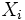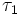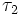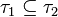# Locally Hausdorff space

## Definition

A topological space$X$ is termed locally Hausdorff if it satisfies the following equivalent conditions:

1. For every point$x \in X$, there is an open subset$U$ of$X$ containing$x$ which is Hausdorff in the subspace topology.
2. For every point$x \in X$, and every open subset$V$ of$X$ containing$x$, there is an open subset$U$ of$X$ contained in$V$, and which is Hausdorff in the subspace topology from$X$.
3.$X$ is a union of open subsets each of which is a Hausdorff space with the subspace topology.
4.$X$ has a basis comprising Hausdorff spaces.
This article defines a property of topological spaces: a property that can be evaluated to true/false for any topological space|View a complete list of properties of topological spaces
This is a variation of Hausdorff space. View other variations of Hausdorff space

## Formalisms

### In terms of the locally operator

This property is obtained by applying the locally operator to the property: Hausdorff space

Note that since Hausdorffness is hereditary, some variants of the locally operator all collapse to the same meaning. In particular, every point being contained in an open Hausdorff subset is equivalent to having a basis of open Hausdorff subsets.

## Metaproperties

Metaproperty name Satisfied? Proof Statement with symbols
box product-closed property of topological spaces Yes local Hausdorffness is box product-closed If$X_i, i \in I$ is a (finite or infinite) collection of locally Hausdorff topological spaces, the product of all the$X_i$s, equipped with the box topology, is also locally Hausdorff.
subspace-hereditary property of topological spaces Yes local Hausdorffness is hereditary Suppose$X$ is a locally Hausdorff space and$A$ is a subset of$X$. Under the subspace topology,$A$ is also locally Hausdorff.
local property of topological spaces Yes (by definition) Suppose$X$ is a locally Hausdorff space and$x \in X$. Then, there exists an open subset$U$ of$X$ containing$x$ such that$U$ is locally Hausdorff.
refining-preserved property of topological spaces Yes localHausdorffness is refining-preserved Suppose$\tau_1$ and$\tau_2$ are two topologies on a set$X$, such that$\tau_1 \subseteq \tau_2$, i.e., every subset of$X$ open with respect to$T_1$ is also open with respect to$\tau_2$. Then, if$X$ is locally Hausdorff with respect to$\tau_1$, it is also locally Hausdorff with respect to$\tau_2$.

## Relation with other properties

### Stronger properties

Property Meaning Proof of implication Proof of strictness (reverse implication failure) Intermediate notions
Hausdorff space Hausdorff implies locally Hausdorff locally Hausdorff not implies Hausdorff (the standard example is the line with two origins) |FULL LIST, MORE INFO